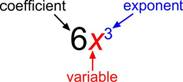## Defining and Evaluating Polynomials

### Learning Outcomes

• Define polynomials
• Evaluate a polynomial for a given value

## Identify the terms, the coefficients, and the exponents of a polynomial

Polynomials are algebraic expressions that are created by combining numbers and variables using arithmetic operations such as addition, subtraction, multiplication, division, and exponentiation. A polynomial expression consists of the sum or difference of terms in which each term is a real number, a variable, or the product of a real number and variable(s) with a non-negative integer exponents. Non negative integers are $0, 1, 2, 3, 4$, …

Polynomials are very useful in applications from science and engineering to business. You may see a resemblance between expressions, which we have been studying in this course, and polynomials.  Polynomials are a special sub-group of mathematical expressions and equations.

The following table is intended to help you tell the difference between what is a polynomial and what is not.

 IS a Polynomial Is NOT a Polynomial Because $2x^2-\frac{1}{2}x -9$ $\frac{2}{x^{2}}+x$ Polynomials only have variables in the numerator $\frac{y}{4}-y^3$ $\frac{2}{y}+4$ Polynomials only have variables in the numerator $\sqrt{12}\left(a\right)+9$ $\sqrt{a}+7$ Variables under a root are not allowed in polynomials

The basic building block of a polynomial is a monomial. When it is of the form $a{x}^{m}$, where $a$ is a constant and $m$ is a whole number, it is called a monomial. A monomial is one term and can be a number, a variable, or the product of a number and variables with an exponent. The number part of the term is called the coefficient.

Examples of monomials:

• number, or coefficient: ${6}$
• variable: ${x}$
• product of coefficient and variable: ${6x}$
• product of coefficient and variable with an exponent: ${6x}^{3}$The coefficient can be any real number, including $0$. The exponent of the variable must be a whole number $—0, 1, 2, 3,$ and so on.  The value of the exponent is the degree of the monomial. Remember that a variable that appears to have no exponent really has an exponent of $1$. And a monomial with no variable has a degree of  $0$. (Since $x^{0}$ has the value of 1 if $x\neq0$, a number such as $3$ could also be written $3x^{0}$, if $x\neq0$ as $3x^{0}=3\cdot1=3$.)  A monomial cannot have a variable in the denominator or a negative exponent.

### Example

Identify the coefficient, variable, and degree of the variable for the following monomial terms:
1) $9$
2) $x$
3) $\displaystyle \frac{3}{5}{{k}^{8}}$

## Evaluate a polynomial for given values of the variable

Previously we evaluated expressions by “plugging in” numbers for variables. Since polynomials are expressions, we’ll follow the same procedures to evaluate polynomials—substitute the given value for the variable into the polynomial, and then simplify.  To evaluate an expression for a value of the variable, you substitute the value for the variable every time it appears. Then use the order of operations to find the resulting value for the expression.

### example

Evaluate $3{x}^{2}-9x+7$ when

1. $x=3$
2. $x=-1$

Solution

 1. $x=3$ $3{x}^{2}-9x+7$ Substitute $3$ for $x$ $3{\left(3\right)}^{2}-9\left(3\right)+7$ Simplify the expression with the exponent. $3\cdot 9 - 9\left(3\right)+7$ Multiply. $27 - 27+7$ Simplify. $7$
 2. $x=-1$ $3{x}^{2}-9x+7$ Substitute $−1$ for $x$ $3{\left(-1\right)}^{2}-9\left(-1\right)+7$ Simplify the expression with the exponent. $3\cdot 1 - 9\left(-1\right)+7$ Multiply. $3+9+7$ Simplify. $19$

### Example

Evaluate $3x^{2}-2x+1$ for $x=-1$.

### Example

Evaluate $\displaystyle -\frac{2}{3}p^{4}+2p^{3}-p$ for $p = 3$.

### try it

The following video presents more examples of evaluating a polynomial for a given value.

The following video provides another example of how to evaluate a quadratic polynomial for a negative number.

### example

The polynomial $-16{t}^{2}+300$ gives the height of an object $t$ seconds after it is dropped from a $300$ foot tall bridge. Find the height after $t=3$ seconds.

## Contribute!

Did you have an idea for improving this content? We’d love your input.